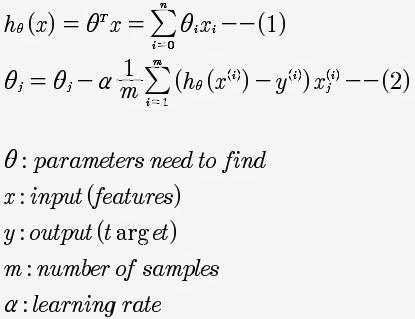## Monday, 11 November 2013

### machine learning--01 : linear regression and batch gradient descent

Linear regression is one of the famous machine learning algorithm.This algorithm assume the relationship between the input(features) and the output(targets) are linear, it use a hypothesis to predict the result.Equation 1 is the hypothesis we use to predict the results, usually we define X0 as 1.To find out the parameters, we could use batch gradient descent.Since the square error produce by linear regression only has one minimum value(it is a convex), we don't need to worry about the starting point.You could find more details on this website. Implement this algorithm with the help of openCV is pretty simple(octave/matlab even more simpler).

```/**
*@brief linear regression
*@param features input sequence
*@param labels output sequence
*@param theta the parameters we want to find
*@return new theta
*/
template<typename T>
cv::Mat_<T> linear_regression(cv::Mat_<T> const &features,
cv::Mat_<T> const &labels,
cv::Mat_<T> const &theta)
{
cv::Mat_<T> result = features.clone();
cv::transpose(result, result);
result *= (features * theta - labels);

return result;
}

/**
*@brief batch gradient descent
*@param features input sequence
*@param labels output sequence
*@param alpha determine the step of each iteration, smaller alpha would
* cause longer time to iterate but with higher chance to converge;
* larger a;pha will run faster but with higher chance to divert.
* Since this function has a fixed iteration time, so alpha only
* affect accuracy.
*@param iterate iterate time
*@return theta, the parameters of batch gradient descent searching
*/
template<typename T>
cv::Mat_<T> batch_gradient_descent(cv::Mat_<T> const &features,
cv::Mat_<T> const &labels,
T alpha = 0.07, size_t iterate = 1)
{
cv::Mat_<T> theta = cv::Mat_<T>::zeros(features.cols, 1);
T const ratio = alpha / features.rows;
for(size_t i = 0; i != iterate; ++i){
cv::Mat_<T> const data = ratio * linear_regression
(features, labels, theta);
theta -= data;
}

return theta;
}
```

If you can't accept the vectorize equation of the function linear_regression, you could expand it as following.